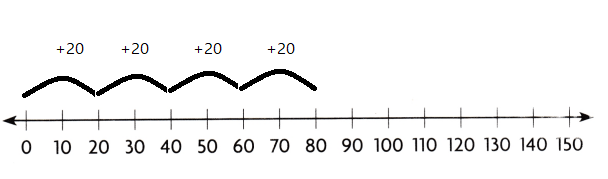Refer to our Texas Go Math Grade 3 Answer Key Pdf to score good marks in the exams. Test yourself by practicing the problems from Texas Go Math Grade 3 Module 9 Assessment Answer Key.

Vocabulary

Choose the best term from the box.

Question 1.
When you multiply the tens and the ones separately and then add the products, you are finding ___. (p. 293)
Partial products
Explanation:
The partial product method involves multiplying each digit of a number in turn with each digit of another where each digit maintains its place.

Concepts and Skills

Use place value to find the product. TEKS 3.4.G

Question 2.
4 × 80 = 4 × __ tens
= __ tens = ____
8 tens = 32 tens = 320
Explanation:
4 × 80 = 4 × 8 tens
= 32 tens = 320

Question 3.
7 × 70 = 7 × __ tens
= ___ tens = ___
7 tens = 49 tens = 490
Explanation:
7 × 70 = 7 × 7 tens
= 49 tens = 490

Find the product. Use models or strategies to help. TEKS 3.4.F, 3.4.G

Fill in the bubble for the correct answer choice.

Question 4.
6 × 40 = ___
Explanation:
6 x 4 tens = 24 tens = 240

Question 5.
7 × 60 = ___
Explanation:
7 x 6 tens = 42 tens = 420

Question 6.
3 × 54 = ___
Explanation:
The product of 3 x 54 = 162

Question 7.
__ = 8 × 37
Explanation:
The product of
8 x 37 = 296

Question 8.Explanation:
The product of
30 and 6  is 180

Question 9.
4 × 20 × 2
Explanation:
The product of
4 x 20 x 2 = 160

Question 10.Explanation:
The product of
18 and 5 is 90

Question 11.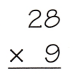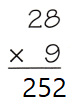Explanation:
The product of
28 and 9 is 252

Question 12.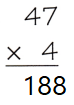Explanation:
The product of
47 and 4 is188

Question 13.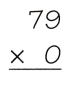Explanation:
The product of
79 and  0 is 0

Question 14.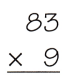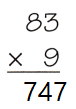Explanation:
The product of
83 and 9 is 747

Question 15.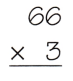Explanation:
The product of
66 and 3 is 198

Texas Test Prep

Fill in the bubble for the correct answer choice.

Question 16.
In Blake’s school, there are 6 third-grade classrooms with 23 students and 1 teacher in each class. How many
third-grade students and teachers are there? TEKS 3.4.G
(A) 138
(B) 144
(C) 30
(D) 124
Explanation:
In Blake’s school, there are 6 third-grade classrooms with 23 students
6 x 23 = 138
and 1 teacher in each class.
138 + 6 = 144

Question 17.
Which equation is an example of the Distributive Property of Multiplication? TEKS 3.4.G
(A) 8 × 20 = 8 × (10 + 10)
(B) 8 × 20 = 20 × 8
(C) 20 × 0 = 0
(D) (8 × 3) × 2 = 8 × (3 × 2)
Explanation:
8 × 20 = 8 × (10 + 10)  is an example of the Distributive Property of Multiplication

Question 18.
Alli put 10 pages of 2 CDs in each of 4 albums. How many CDs did she put in the albums? TEKS 3.4.G
(A) 16
(B) 80
(C) 40
(D) 20
Explanation:
Alli put 10 pages of 2 CDs
2 x 10 = 20
in each of 4 albums.
20 x 4 = 80 CD’s  she put in the albums.

Question 19.
Use the number line to find 4 × 20. What is the product? TEKS 3.4.G
Record your answer and fill in the bubbles on the grid. Be sure to use the correct place value.